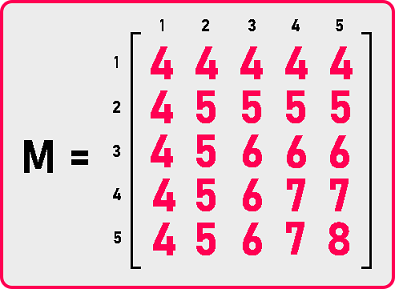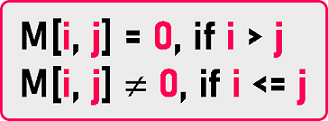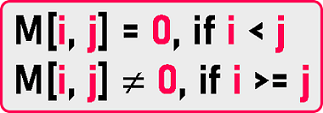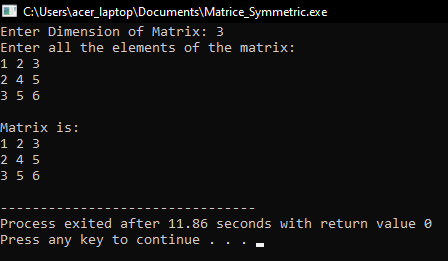# Symmetric Matrix

## Symmetric Matrix in C Language with Examples:

In this article, I am going to discuss Symmetric Matrix in C Language with Examples. Please read our previous article, where we discussed Upper Triangular Matrix by Column-Major Mapping in C and C++ Language with Examples.

##### Symmetric Matrix:

In this article, we will see the implementation of the symmetric Matrix.Here we have taken an example of a symmetric matrix of order 5×5. Let us observe the property of these metrics. Let ‘i’ and ‘j’ represent row number and column number of the above-given matrix.

Let’s take the example of M  and M . As we can see in the matrix both the elements have the same value of ‘4’. Let’s take another example of M  and M . Again, both the elements have the same value of ‘6’. So, if you observe the elements at the location of (i, j) are equal to the elements of location (j, i).This is the property of a symmetric matrix. Do we have to store all the elements of a symmetric matrix? No. If we store (i, j) value then we can retrieve (j, i) also. So, if we know any element that (i, j) then also we know the element of (j, i) because they are the same. So, it means it’s sufficient to store either elements in the lower triangle or the elements in the upper triangle.

Those elements would be sufficient. We can store the matrix by taking only upper triangular part or lower triangular part. We know that:

###### For Upper Triangular part:Index (M [i][j]) = [ j * (j – 1) / 2] + (i – 1)

###### For Lower Triangular part:Index (M [i][j]) = [i * (i – 1) / 2] + (j-1)

We have discussed these two in our previous articles. So, you can check there for a detailed explanation.

##### Symmetric Matrix Code in C Language:
```#include <stdio.h>
#include <stdlib.h>

struct Matrix
{
int *B;
int n;
};

void Set(struct Matrix *m, int i, int j, int y)
{
if (i >= j)
m->B[i * (i - 1) / 2 + j - 1] = y;
}

int Get(struct Matrix m, int i, int j)
{
if (i >= j)
return m.B[i * (i - 1) / 2 + j - 1];
else
return 0;
}

void Display(struct Matrix m)
{
int i, j;

printf ("\nMatrix is: \n");

for (i = 1; i <= m.n; i++)
{
for (j = 1; j <= m.n; j++)
{
if (i >= j)
printf ("%d ", m.B[i * (i - 1) / 2 + j - 1]);
if (j > i)
printf ("%d ", m.B[j * (j - 1) / 2 + i - 1]);
}
printf ("\n");
}
}

int main()
{
struct Matrix M;
int i, j, y;

printf ("Enter Dimension of Matrix: ");
scanf ("%d", &M.n);
M.B = (int *) malloc (M.n * (M.n + 1) / 2 * sizeof (int));

printf ("Enter all the elements of the matrix:\n");
for (i = 1; i <= M.n; i++)
{
for (j = 1; j <= M.n; j++)
{
scanf ("%d", &y);
Set(&M, i, j, y);
}
}
Display (M);
}
```
###### Output:In the next article, I am going to discuss TriDiagonal and TriBand Matrix in C Language with Examples. Here, in this article, I try to explain Symmetric Matrix in C Language with Examples and I hope you enjoy Symmetric Matrix in C Language with Examples article.